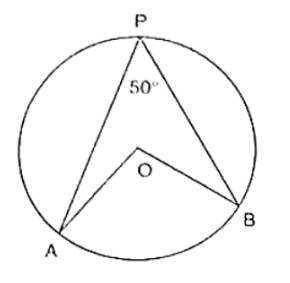# In figure, O is the centre of the circle.

Question:

In figure, O is the centre of the circle. If ∠APB = 50°, find ∠AOB and ∠OAB.Solution:

∠APB = 50°

By degree measure theorem

∠AOB = 2∠APB

⇒ ∠APB = 2 × 50° = 100° since OA = OB [Radius of circle]

Then ∠OAB = ∠OBA [Angles opposite toequalsides]

Let ∠OAB = x

In ΔOAB, by angle sum property

∠OAB + ∠OBA + ∠AOB = 180°

⟹ x + x + 100° = 180°

⟹ 2x = 180° - 100°

⟹ 2x = 80°

⟹ x = 40°

∠OAB = ∠OBA = 40°